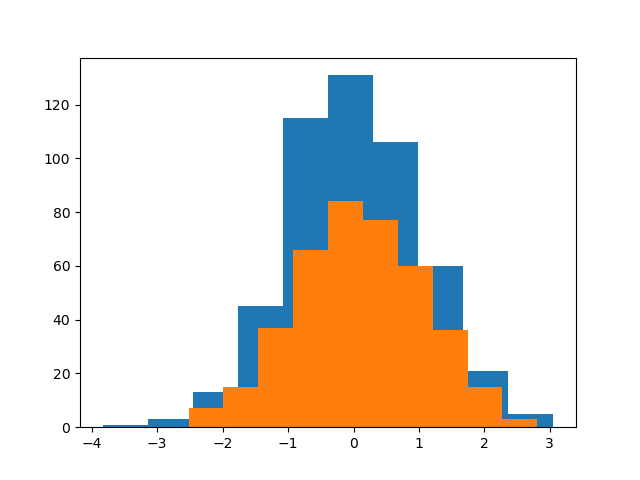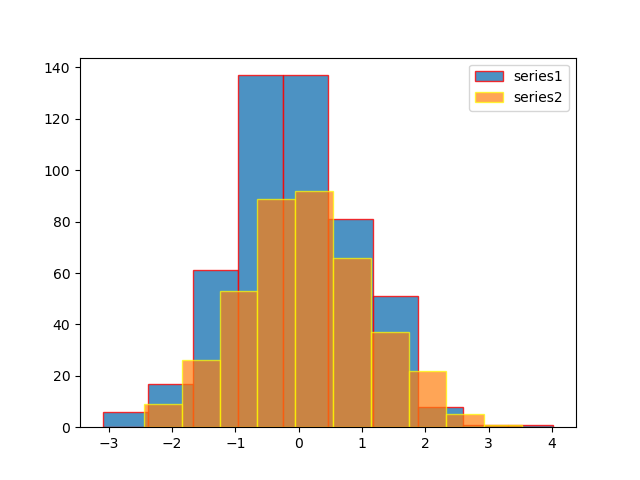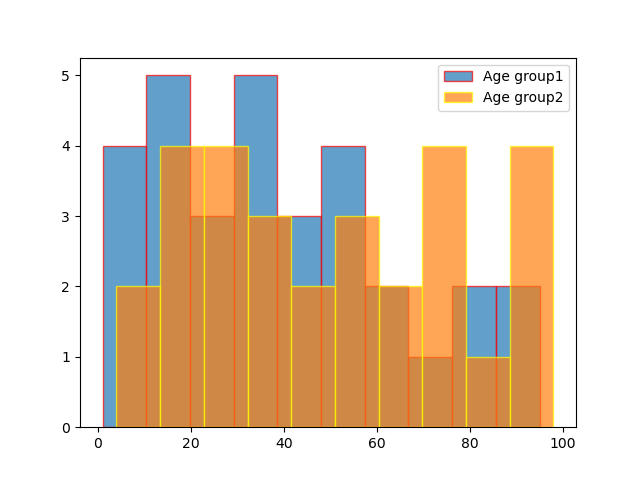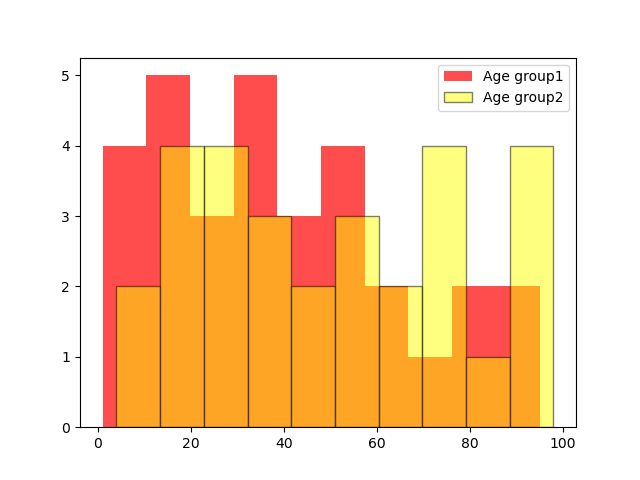# How to plot two histograms together in Matplotlib?

• Difficulty Level : Expert
• Last Updated : 12 Sep, 2022

Creating the histogram provides the visual representation of data distribution. By using a histogram we can represent a large amount of data and its frequency as one continuous plot.

## How to plot a histogram using Matplotlib

For creating the Histogram in Matplotlib we use hist() function which belongs to pyplot module. For plotting two histograms together, we have to use hist() function separately with two datasets by giving some settings.

### Syntax of matplotlib.pyplot.hist

matplotlib.pyplot.hist(x, bins, edgecolor color, label)

Example 1:

Here, we are simply taking two series using the Numpy random and passing both series to the hist()function, and we’re using the same plot to plot two histograms together.

## Python3

 `# importing libraries` `import` `matplotlib.pyplot as plt` `import` `numpy as np`   `# generating two series of random ` `# values using numpy random module ` `# of shape (500,1)` `series1 ``=` `np.random.randn(``500``, ``1``)` `series2 ``=` `np.random.randn(``400``, ``1``)`   `# plotting first histogram` `plt.hist(series1)`   `# plotting second histogram` `plt.hist(series2)`   `# Showing the plot using plt.show()` `plt.show()`

Output:Example 2:

Here, we are using label, edgecolor, and opacity.

## Python3

 `# importing libraries` `import` `matplotlib.pyplot as plt` `import` `numpy as np` `from` `numpy.lib.histograms ``import` `histogram`   `# generating two series of random values ` `# using numpy random module of shape (500,1)` `series1 ``=` `np.random.randn(``500``, ``1``)` `series2 ``=` `np.random.randn(``400``, ``1``)`   `# plotting first histogram` `plt.hist(series1, label``=``'series1'``, alpha``=``.``8``, edgecolor``=``'red'``)`   `# plotting second histogram` `plt.hist(series2, label``=``'series2'``, alpha``=``0.7``, edgecolor``=``'yellow'``)` `plt.legend()`   `# Showing the plot using plt.show()` `plt.show()`

Output:Example 3:

Histograms represent two age groups using given data.

## Python3

 `# importing libraries` `import` `matplotlib.pyplot as plt`   `# giving two age groups data` `age_g1 ``=` `[``1``, ``3``, ``5``, ``10``, ``15``, ``17``, ``18``, ``16``, ``19``,` `          ``21``, ``23``, ``28``, ``30``, ``31``, ``33``, ``38``, ``32``, ` `          ``40``, ``45``, ``43``, ``49``, ``55``, ``53``, ``63``, ``66``, ` `          ``85``, ``80``, ``57``, ``75``, ``93``, ``95``]`   `age_g2 ``=` `[``6``, ``4``, ``15``, ``17``, ``19``, ``21``, ``28``, ``23``, ``31``, ` `          ``36``, ``39``, ``32``, ``50``, ``56``, ``59``, ``74``, ``79``, ``34``, ` `          ``98``, ``97``, ``95``, ``67``, ``69``, ``92``, ``45``, ``55``, ``77``,` `          ``76``, ``85``]`   `# plotting first histogram` `plt.hist(age_g1, label``=``'Age group1'``, bins``=``14``, alpha``=``.``7``, edgecolor``=``'red'``)`   `# plotting second histogram` `plt.hist(age_g2, label``=``"Age group2"``, bins``=``14``, alpha``=``.``7``, edgecolor``=``'yellow'``)` `plt.legend()`   `# Showing the plot using plt.show()` `plt.show()`

Output:Example 4

Changing bar color from the default

## Python3

 `# importing libraries` `import` `matplotlib.pyplot as plt`   `# giving two age groups data` `age_g1 ``=` `[``1``, ``3``, ``5``, ``10``, ``15``, ``17``, ``18``, ``16``, ``19``, ``21``,` `          ``23``, ``28``, ``30``, ``31``, ``33``, ``38``, ``32``, ``40``, ``45``, ` `          ``43``, ``49``, ``55``, ``53``, ``63``, ``66``, ``85``, ``80``, ``57``, ` `          ``75``, ``93``, ``95``]`   `age_g2 ``=` `[``6``, ``4``, ``15``, ``17``, ``19``, ``21``, ``28``, ``23``, ``31``, ``36``,` `          ``39``, ``32``, ``50``, ``56``, ``59``, ``74``, ``79``, ``34``, ``98``, ``97``,` `          ``95``, ``67``, ``69``, ``92``, ``45``, ``55``, ``77``, ``76``, ``85``]`   `# plotting first histogram` `plt.hist(age_g1, label``=``'Age group1'``, alpha``=``.``7``, color``=``'red'``)`   `# plotting second histogram` `plt.hist(age_g2, label``=``"Age group2"``, alpha``=``.``5``,` `         ``edgecolor``=``'black'``, color``=``'yellow'``)` `plt.legend()`   `# Showing the plot using plt.show()` `plt.show()`

Output:My Personal Notes arrow_drop_up
Recommended Articles
Page :